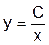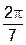More Favorite Geometry Proofs   Saturday, August 9, 2014, 1 PM – 4:20 PM Hilton Portland, Ballroom Level, Galleria I   Organizer:  Sarah Mabrouk, Framingham State University This session invites presenters to share their favorite undergraduate geometry proofs. These proofs should be suitable for Euclidean and non-Euclidean geometry courses as well as for courses frequently referred to as "modern" or "higher" geometry but not those related to differential geometry or (low-level) graduate courses. Proofs must be for theorems other than the Pythagorean Theorem and should be different from those presented during the MAA MathFest 2013 paper session (see http://www.framingham.edu/~smabrouk/Maa/mathfest2013/ for more information). Presenters must do the full proof, discuss how the proof fits into the course, provide information regarding prerequisite topics for the proof, and discuss associated areas with which students have difficulty and how such concerns are addressed so that students understand the proof. Presenters are invited to discuss how they have modified the proof over time as well as to share historical information for "classic" proofs and explorations/demonstrations that they use to help students understand the associated theorem.  Abstracts should include the theorem to be proved/discussed as well as brief background information.

 1 PM -  1:15 PM A Proof of Ptolemy’s Theorem via Inversions Deirdre Longacher Smeltzer, Eastern Mennonite University   Ptolemy's theorem, attributed to second century Greek mathematician Claudius Ptolemaeus, gives necessary and sufficient conditions for one to be able to inscribe a given quadrilateral in a circle.  A standard proof involves using inscribed angles and similar triangles. A more elegant and modern proof utilizes an inversion in the plane and resulting properties to establish a generalization of the theorem. 1:20 PM -  1:35 PM Archimedes’ Twin Circles in an Arbelos Dan C. Kemp, South Dakota State University   Proposition 5 from Archimedes’ Book of Lemmas was popularized in 1954 by Leon Bankoff as a surprise in an arbelos.  An arbelos consists of three mutually tangent semicircles with diameters on a common line and lying on the same side of that line. Archimedes asserts that in an arbelos, the two circles that are tangent to two of the semicircles and the common tangent of the smaller semicircles are congruent.   Archimedes’ synthetic proof, suitable for presentation in a geometry course, will be given. Archimedes’ proof is historically interesting because it contains the first known reference (‘... by the properties of triangles…’) of the altitudes of a triangle being concurrent. Also a modern proof using analytic geometry will be presented. If time permits, further discussion of the twin circles of Archimedes will be given. 1:40 PM -  1:55 PM Euler’s Famous Line: Gateway to The Harmonic 2:1 Centroid Concurrency Alvin Swimmer, Arizona State University   It was Archimedes of Syracuse (287-212 b.c.) that first proved that the three medians of every triangle are concurrent at the centroid, G, which divides each median in the ratio 2:1.  Two millenia later, in 1763, Leonhard Euler, the most prolific mathematician of all time, discovered the line determined by the orthocenter, O, and the circumcenter C, of any (non-equilateral) triangle also contains the centroid G which divides the interval [O,C] in the ratio 2:1.   In more recent times, Tom Apostle and Mamikon Mnatsakian, discovered in 2004, that the incenter B and the 1-dimensional center of mass \$D\$ determine a line which contains the centroid and G divides the interval [B,D] in the same 2:1 ratio.  In 2006, it became clear to me that, the 5 lines and intervals mentioned above, associated with each (non-equilateral) triangle are part of an infinite family of lines all concurrent at G, Each of these lines is determined by 2 points which determine an interval divided by G, in the 2:1 ratio.  I call this family The Harmonic 2:1 Centroid Currency. 2 PM -  2:15 PM Reflections in Geometry David Marshall, Monmouth University   Our junior level geometry course provides a study of Euclidean and non-Euclidean geometries, but leaves much of the specific content up to the instructor, and the variation can be large. My course emphasizes transformations, starting with the classification of isometries of the Euclidean plane.  The Three-Reflections Theorem plays a central role in this classification and helps with several other pedagogical and content goals.  It provides students with an opportunity to (1) seek generalizations later in the course, (2) experiment with a “proof by generic example”, (3) make good practical use of available technology, and (4) do a little group theory.  The theorem (and related results) also serves as an entry way into more general discussions of classification theorems, Klein's Erlanger Program, and the role of group theory in geometry. 2:20 PM -  2:35 PM Reflections on Reflections Thomas Q. Sibley, St. John's University   The Common Core State Standards emphasize geometric transformations, highlighting the Three Mirror Theorem: Every Euclidean plane isometry is the composition of at most three mirror reflections. The proofs for this theorem and the theorems leading to it also generalize beautifully to hyperbolic and spherical geometries.  Even more, they generalize to higher dimensions for all three of these geometries with only minor modifications.  Future secondary teachers, indeed all mathematics majors, can gain important insight by considering these theorems from this unifying perspective. 2:40 PM -  2:55 PM The Shortest Path Between Two Points and a Line Justin Allen Brown, Olivet Nazarene University   In a geometry classroom, asking the question in the open-ended form above often leads to interesting ideas from students.  Many of their ideas do not yield the shortest path in general, but result in an interesting discussion.  And once students realize that their initial idea is incorrect, they are invested in finding or at least seeing the correct proof.  We will discuss some of these incorrect ideas, as well as the proof of the theorem, which uses similar triangles. 3 PM -  3:15 PM The Perfect Heptagon from the Square Hyperbola   It is well known that the perfect 7-sided heptagon cannot be constructed with a compass and a straight edge alone, but it can be done with an angle trisector. However, virtually all perfect heptagon constructions include a “magic” step, which presents some angle 3q, followed immediately by presenting the angle q, then continuing on with the construction.  It is also well known that angle trisection cannot be done with a compass and a straight edge alone because it requires solving an irreducible cubic, but that it can be done with the help of a non-circular conic section.  The first conic section we usually learn about is the inverse relationshipwhich makes it a nice item to use for implementing a trisection construction within a heptagon construction. Starting with an unmarked xy-coordinate system and a single square hyperbola H, we show that adding 4 circles and 10 lines gives us theangle for the perfect heptagon. The proof of why it works and the motivation for how a square hyperbola naturally arises provide a nice example of combining both constructive geometry and elementary trigonometry. 3:20 PM – 3:35 PM The Many Shapes of Hyperbolas in Taxicab Geometry Ruth I. Berger, Luther College   Taxicab geometry is a good topic for open-ended explorations in non-Euclidean geometry. My undergraduate students are always surprised at the many different looking hyperbolas they discover.  A simple geometric argument can be given to classify the different hyperbola shapes, as well as the other conic sections.  The shape depends on the slope of the line connecting the foci.  The underlying reason for this is that in taxicab geometry circles have sides of slope 1 and -1. 3:40 PM – 3:55 PM Geometry Knows Topology: The Gauss-Bonnet Theorem Jeff Johannes, SUNY Geneseo   Last year I spoke in this session about angle sum of spherical triangles. And I think now “I cannot have two favorite geometry theorems.”  On the other hand, what if we think of geometry in a different context? What if we work with surfaces of non-constant curvature for a change?  Now curvature is a variable quantity. We cannot speak any longer of the simple situation of the sphere, but instead we talk about a more sophisticated view of changing geometry.  In this context, we cannot ask what the curvature of the whole surface is, but we can consider the integral of the curvature over the entire surface.  From this perspective we can zoom out from those triangles with their angle sums and see global topological properties of our surface.  In this talk we will move from angle sum through a sequence of generalizations and new ideas to find the famous and powerful Gauss-Bonnet Theorem. 4 PM – 4:15 PM Finding the Fermat Point by Physics and by Transformation Philip Todd, Saltire Software   My new favorite way of finding the point with minimum sum of distances to the three vertices of a triangle involves a mechanical thought computer described in Mark Levi’s “The Mathematical Mechanic”.  An interactive model of this mechanical system can motivate the conjecture that for some triangles the solution lies at the point which subtends equal angles to the sides.  It can also motivate accurate conjectures on conditions for this solution to apply.  An alternative exploration tool for students with no physics background will also be presented.  Again, this will allow for both the solution and the conditions under which the solution applies to be induced.   When minimizing the length of a path, I like proofs which apply transforms to create a path equal to the one to be minimized, but where the minimal path is clearly a straight line.  We can use reflections in this way to solve Fagnano’s problem of finding the inscribed triangle with minimal.  Can we apply transforms to reduce the Fermat Toricelli Point problem to finding the shortest distance between two points?  Our conjecture suggests that 120 degree rotations may be the transforms of choice.  Application of these rotations yields a visual proof both of the result for triangles which have no vertices over 120 degrees, and of its breakdown when there are angles greater than 120 degrees.

This page was created and is maintained by S. L. Mabrouk, Framingham State University.